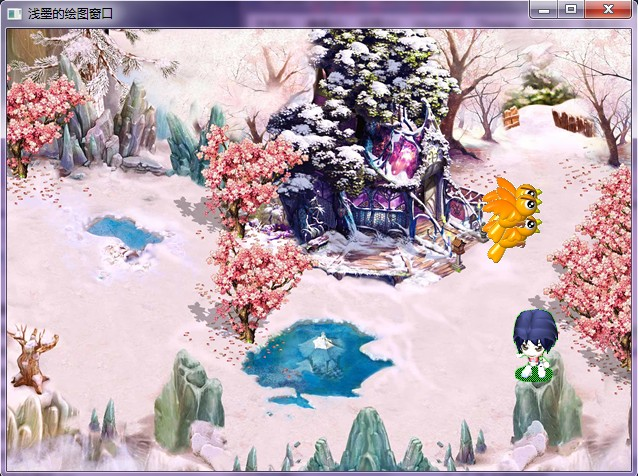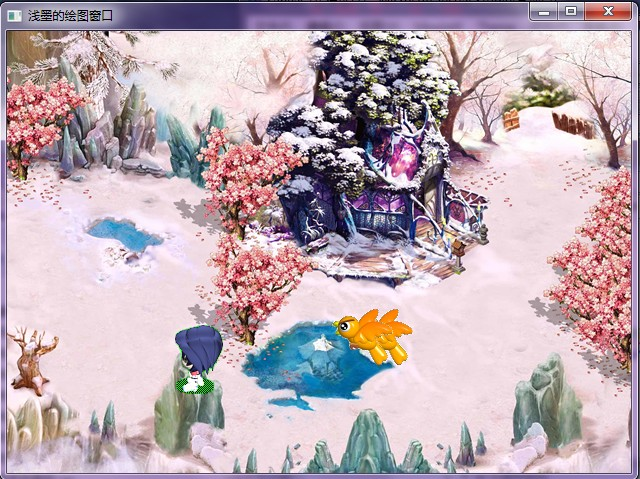【Visual C++】游戏开发笔记十五 游戏人工智能（一） 运动型游戏AI

年薪达不到25W大数据工程师、拿不到Offer全额退款->>><1>追逐移动

【算法1】

If（枭兽X>幻影刺客X）

else

If（枭兽Y<幻影刺客Y）

else

【算法2】

If（枭兽HP>200）              //生命值大于200时才追
(
P=rand()%3;                   //取随机数除以3的余数
If(p!=1)                        //余数不为1时进行追逐
{
If（枭兽X>幻影刺客X）

else

If（枭兽Y<幻影刺客Y）

else

}
else
枭兽HP+=5           //怪物不动，自动补5点血
）

<2>躲避移动

【算法3】

If（枭兽X>幻影刺客X）

else

If（枭兽Y<幻影刺客Y）

else

#include "stdafx.h"
#include <stdio.h>

//全局变量声明
HINSTANCE hInst;
HBITMAP girl,bg,bird;
HDC		hdc,mdc,bufdc;
HWND	hWnd;

DWORD	tPre,tNow,nowX,nowY;
POINT	p;               //用于记录3只小鸟的贴图坐标
int		num,dir,x,y;       //x，y变量为人物贴图坐标，dir为人物移动方向，这里我们中以0，1，2，3代表人物上，下，左，右方向上的移动：num为连续贴图中的小图编号

//全局函数声明
ATOM				MyRegisterClass(HINSTANCE hInstance);
BOOL				InitInstance(HINSTANCE, int);
LRESULT CALLBACK	WndProc(HWND, UINT, WPARAM, LPARAM);
void				MyPaint(HDC hdc);

//****WinMain函数，程序入口点函数***********************
int APIENTRY WinMain(HINSTANCE hInstance,
HINSTANCE hPrevInstance,
LPSTR     lpCmdLine,
int       nCmdShow)
{
MSG msg;

MyRegisterClass(hInstance);

//初始化
if (!InitInstance (hInstance, nCmdShow))
{
return FALSE;
}

GetMessage(&msg,NULL,NULL,NULL);            //初始化msg
//消息循环
while( msg.message!=WM_QUIT )
{
if( PeekMessage( &msg, NULL, 0,0 ,PM_REMOVE) )
{
TranslateMessage( &msg );
DispatchMessage( &msg );
}
else
{
tNow = GetTickCount();
if(tNow-tPre >= 40)
MyPaint(hdc);
}
}

return msg.wParam;
}

//****设计一个窗口类，类似填空题，使用窗口结构体*******************
ATOM MyRegisterClass(HINSTANCE hInstance)
{
WNDCLASSEX wcex;

wcex.cbSize = sizeof(WNDCLASSEX);
wcex.style			= CS_HREDRAW | CS_VREDRAW;
wcex.lpfnWndProc	= (WNDPROC)WndProc;
wcex.cbClsExtra		= 0;
wcex.cbWndExtra		= 0;
wcex.hInstance		= hInstance;
wcex.hIcon			= NULL;
wcex.hCursor		= NULL;
wcex.hCursor		= LoadCursor(NULL, IDC_ARROW);
wcex.hbrBackground	= (HBRUSH)(COLOR_WINDOW+1);
wcex.lpszClassName	= "canvas";
wcex.hIconSm		= NULL;

return RegisterClassEx(&wcex);
}

//****初始化函数*************************************
// 加载位图并设定各种初始值
BOOL InitInstance(HINSTANCE hInstance, int nCmdShow)
{
HBITMAP bmp;
hInst = hInstance;

hWnd = CreateWindow("canvas", "浅墨的绘图窗口" , WS_OVERLAPPEDWINDOW,
CW_USEDEFAULT, 0, CW_USEDEFAULT, 0, NULL, NULL, hInstance, NULL);

if (!hWnd)
{
return FALSE;
}

MoveWindow(hWnd,10,10,640,480,true);
ShowWindow(hWnd, nCmdShow);
UpdateWindow(hWnd);

hdc = GetDC(hWnd);
mdc = CreateCompatibleDC(hdc);
bufdc = CreateCompatibleDC(hdc);

//建立空的位图并置入mdc中
bmp = CreateCompatibleBitmap(hdc,640,480);
SelectObject(mdc,bmp);

//设定人物贴图初始位置和移动方向
x = 300;
y = 250;
dir = 0;
num = 0;
nowX = 300;
nowY = 300;

//载入各连续移动位图及背景图

p.x = 30;
p.y = 100;

p.x = 250;
p.y = 250;

p.x = 500;
p.y = 400;

MyPaint(hdc);

return TRUE;
}

//****自定义绘图函数*********************************
// 1.人物贴图坐标修正及窗口贴图
//进行AI行为判断并贴图
void MyPaint(HDC hdc)
{
int w,h,i;

//先在mdc中贴上背景图
SelectObject(bufdc,bg);
BitBlt(mdc,0,0,640,480,bufdc,0,0,SRCCOPY);

//按照目前的移动方向取出对应人物的连续走动图，并确定截取人物图的宽度与高度
SelectObject(bufdc,girl[dir]);
switch(dir)
{
case 0:
w = 55;
h = 74;
break;
case 1:
w = 53;
h = 77;
break;
case 2:
w = 60;
h = 74;
break;
case 3:
w = 60;
h = 74;
break;
}
//按照目前的X，Y的值在mdc上进行透明贴图，然后显示在窗口画面上
BitBlt(mdc,x,y,w,h,bufdc,num*w,h,SRCAND);
BitBlt(mdc,x,y,w,h,bufdc,num*w,0,SRCPAINT);

//贴出鸟的图片
SelectObject(bufdc,bird);

for(i=0;i<3;i++)
{

if(rand()%3 != 1)		//有2/3几率进行追踪
{
if(p[i].y > y-16)
p[i].y -= 5;
else
p[i].y += 5;

if(p[i].x > x-25)
p[i].x -= 5;
else
p[i].x += 5;
}

if(p[i].x > x-25)    //判断小鸟的移动方向，从而选择合适的位图朝向
{
BitBlt(mdc,p[i].x,p[i].y,61,61,bufdc,61,61,SRCAND);
BitBlt(mdc,p[i].x,p[i].y,61,61,bufdc,0,61,SRCPAINT);
}
else
{
BitBlt(mdc,p[i].x,p[i].y,61,61,bufdc,61,0,SRCAND);
BitBlt(mdc,p[i].x,p[i].y,61,61,bufdc,0,0,SRCPAINT);
}
}

BitBlt(hdc,0,0,640,480,mdc,0,0,SRCCOPY);

tPre = GetTickCount();         //记录此次绘图时间

num++;
if(num == 8)
num = 0;

}

//****消息处理函数***********************************
// 1.按下【Esc】键结束程序
// 2.按下方向键重设贴图坐标
LRESULT CALLBACK WndProc(HWND hWnd, UINT message, WPARAM wParam, LPARAM lParam)
{
switch (message)
{
case WM_KEYDOWN:	     //按下键盘消息
//判断按键的虚拟键码
switch (wParam)
{
case VK_ESCAPE:           //按下【Esc】键
PostQuitMessage( 0 );  //结束程序
break;
case VK_UP:				  //按下【↑】键
//先按照目前的移动方向来进行贴图坐标修正，并加入人物往上移动的量（每次按下一次按键移动10个单位），来决定人物贴图坐标的X与Y值，接着判断坐标是否超出窗口区域，若有则再次修正
switch(dir)
{
case 0:
y -= 10;
break;
case 1:
x -= 1;
y -= 8;
break;
case 2:
x += 2;
y -= 10;
break;
case 3:
x += 2;
y -= 10;
break;
}
if(y < 0)
y = 0;
dir = 0;
break;
case VK_DOWN:			  //按下【↓】键
switch(dir)
{
case 0:
x += 1;
y += 8;
break;
case 1:
y += 10;
break;
case 2:
x += 3;
y += 6;
break;
case 3:
x += 3;
y += 6;
break;
}

if(y > 375)
y = 375;
dir = 1;
break;
case VK_LEFT:			  //按下【←】键
switch(dir)
{
case 0:
x -= 12;
break;
case 1:
x -= 13;
y += 4;
break;
case 2:
x -= 10;
break;
case 3:
x -= 10;
break;
}
if(x < 0)
x = 0;
dir = 2;
break;
case VK_RIGHT:			   //按下【→】键
switch(dir)
{
case 0:
x += 8;
break;
case 1:
x += 7;
y += 4;
break;
case 2:
x += 10;
break;
case 3:
x += 10;
break;
}
if(x > 575)
x = 575;
dir = 3;
break;
}
break;
case WM_DESTROY:			    	//窗口结束消息
int i;

DeleteDC(mdc);
DeleteDC(bufdc);
for(i=0;i<4;i++)
DeleteObject(girl[i]);
DeleteObject(bg);
ReleaseDC(hWnd,hdc);

PostQuitMessage(0);
break;
default:							//其他消息
return DefWindowProc(hWnd, message, wParam, lParam);
}
return 0;
}——————————浅墨于2012年4月7日